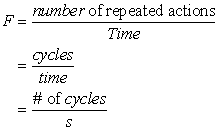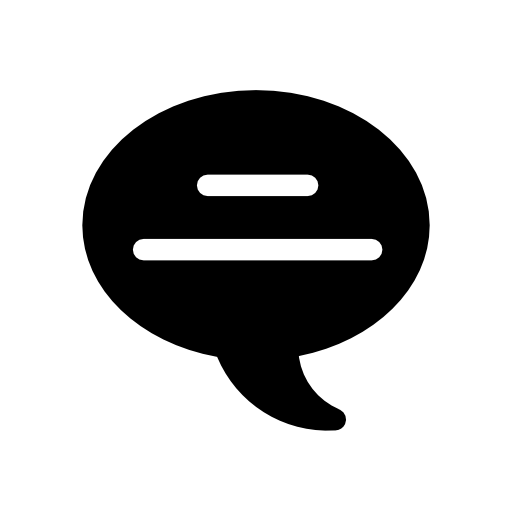Time can be defined as a measure of elapsed regular intervals based on natural recurring events such as the changing of the seasons, sunrise-sunsets, full moon-new moon, high tide and low tide.

Time is a fundamental scientific quantity.

The symbol for time in the metric system is t, and the standard unit is the second; (s) is the symbol for second.

One complete occurrence of a time interval is known as a cycle.

Frequency is a measure of how often cycles are repeated.  Mathematically speaking frequency is the number of repeated actions that occur in a given time. The symbol for Frequency is f and the mathematical formula for frequency is:Note that in the above formula,   " the # of cycles" is actually a "counted quantity" and not a measured quantity therefore cycles, like all quantities that are counted, do not have units.  The assigned unit of Frequency in the metric system (SI Units) is the Hertz [Hz - for short].

[For practical reasons you may want to think of the Hz as being equal to 1/s or s-1]

Example:  What is the frequency of a yo-yo which is being constantly rolled up and down a total of 300 times in 3 minutes?

Solution:

Given: # of cycles = 300;
time = 3 min = 3 min  x 60 s/min = 180 s

Find: f

f = cycles/time  = 300 cycles / 180 s = 1.7 H

Period is the reciprocal of frequency.  The period of a repeated motion is the time it takes to complete one entire cycle.

The symbol for period is T (capital letter T) and the unit for period is the second (s).  In equation form we can express period as:

Example:  What is the period of an ocean wave if on average 20 waves are seen striking the shore in 7 seconds.

Given: # of waves = 20; time = 7 s

Find: T

T= time/cycles = 7 s / 20 waves  = 0.35 s[Note that "# of waves" is also a counted quantity therefore it does not have units].